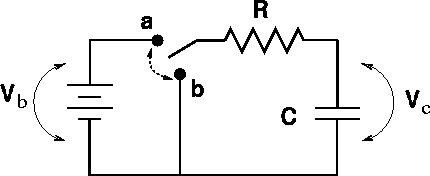RC Circuits Examples

Example #1

The circuit below has been in position a for a long time. At time t = 0 the switch is thrown to position b. DATA: Vb = 12 V, C = 10 mF, R = 20 Wa.) What is the curnent through the resistor just BEFORE the switch is thrown?

I = 0

b.) What is the current through the resistor just AFTER the switch is thrown?

Solution: I = V/R

I = 0.6 amps

c.) What is the charge across the capacitor just BEFORE the switch is thrown?

Solution: Q = CV

Q = 120 mC

d.) What is the charge on the capacitor just AFTER the switch is thrown?

Solution: Charge does not change instantaneously.

Q = 120 mC

e.) What is the charge on the capacitor at at time t = 0.3 msec after the switch is thrown?

Solution: Q = Q0exp(-t/t) , where t = RC = 0.2 msec

Q = 26.8 mC

Example #2

Considering the same circuit, only with the switch thrown from b to a at time t = 0 after having been in position b for a long time. DATA: Vb = 12 V, C = 10 mF, R = 20 Wa.) What is the curnent through the resistor just BEFORE the switch is thrown?

I = 0

b.) What is the current through the resistor just AFTER the switch is thrown?

Solution: I = V/R

I = 0.6 amps

c.) What is the charge across the capacitor just BEFORE the switch is thrown?

Solution: Q = CV

Q = 0

d.) What is the charge on the capacitor just AFTER the switch is thrown?

Solution: Charge does not change instantaneously.

Q = 0

e.) What is the charge on the capacitor at at time t = 0.3 msec after the switch is thrown?

Solution: Q = Q0(1.0 - exp(-t/t)) , where t = RC = 0.2 msec

Q = 93.2 mC